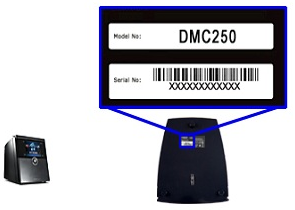Troubleshooting often requires you to know your device’s model, version, MAC address, and serial number.  Aside from the device itself, the model, MAC address, and the serial number of some Linksys devices are also printed on the box.  Select a product category below to know how to find the model, MAC address, and the serial number of your Linksys device.

NOTE:  Depending on the model number, your product may look different from the examples provided below

Directions

VELOP

Step 1:
Flip the node over.

Step 2:
You can find the model, MAC address, and serial number below the Linksys logo.
If there is no version number beside the model number, this means it is version 1.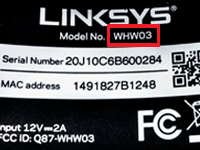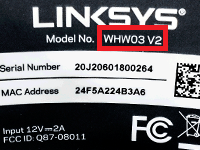EA and E-Series

Step 1:
Look at the top of the device.

Step 2:

You can find the model number below or next to the Linksys logo.  If there is no version number beside the model number, this means it is version 1.  In the example below, the model number of the device is EA9500 V2.

NOTE:  The serial number and the MAC address are located on the sticker underneath the device.  For E3200, you can find the model number on the front panel of the device.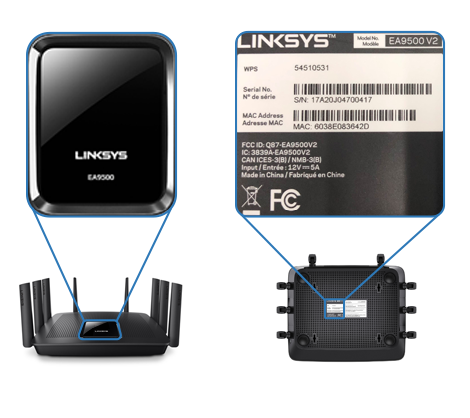Blue and Black

Step 1:
Flip the device over.

Step 2:

You can find the model, MAC address, and serial number on the sticker underneath the device.  If there is no version number beside the model number, this means it is version 1.  In the example below, the model number of the device is WRT54G ver.6.

NOTE:  For WRT1900AC, the model number is located on the front panel, below the Linksys logo.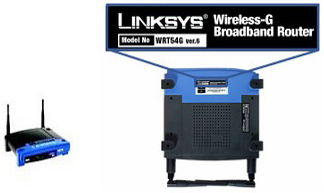Silver

Step 1:
Look at the back panel of the router when it is standing vertically.

Step 2:

You can find the model and serial number on the sticker at the back.  If there is no version number beside the model number, this means it is version 1.  In the example below, the model number of the device is WRT54GX4.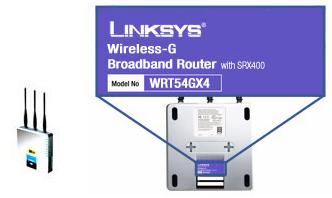Wireless Routers with Voice Ports

Step 1:
Look at the back panel of the router when it is standing vertically.

Step 2:

You can find the model and serial number on the sticker at the back.  If there is no version number beside the model number, this means it is version 1.  In the example below, the model number is WRTP54G V.2.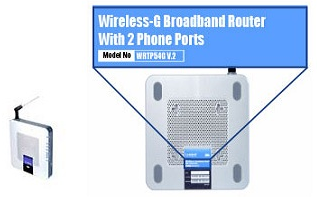Wireless VPN Routers

Step 1:
Look at the back panel of the router when it is standing vertically.

Step 2:
You can find the model and serial number on the sticker at the back.  If there is no version number beside the model number, this means it is version 1.  In the example below, the model number is WRV54G.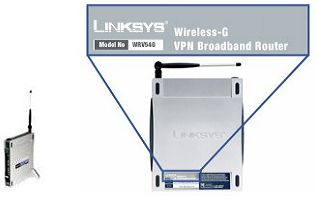Wireless Access Points

Step 1:
Look at the front panel.

Step 2:

You can find the model number below the Linksys logo.
If there is no version number beside the model number, this means it is version 1.  In the example below, the model number is WAP610N.

NOTE:
The MAC address and the serial number are located on the sticker underneath or at the back of the device.  For older Linksys wireless access points, you can find the model number underneath the device.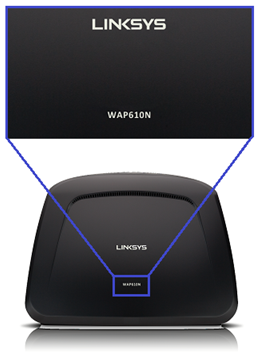Step 1:
Look at the top of the device.

Step 2:

You can find the model number below the Linksys logo.  If there is no version number beside the model number, this means it is version 1.  In the example below, the model number is RE3000W.

NOTE:  The serial number is located on the sticker underneath the device.  For older Linksys wireless range extenders or expanders, you can find the model number underneath the device.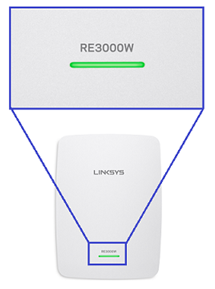PC Card or Card Bus

Step 1:

Step 2:

You can find the model, MAC address, and serial number on the device itself.  If there is no version number beside the model number, this means it is version 1.  In the example below, the model number is WPC54G ver. 7.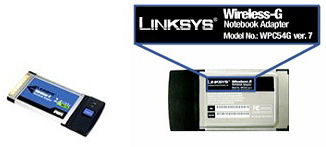Step 1:

Step 2:

You can find the model and serial number on the device itself.
If there is no version number beside the model number, this means it is version 1.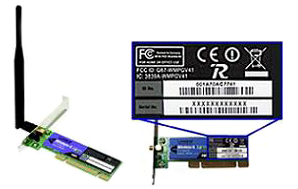Step 1:
You can find the model, MAC address, and serial number on the sticker underneath or at the back of the device.  If there is no version number beside the model number, this means it is version 1.  In the example below, the model number is AE6000.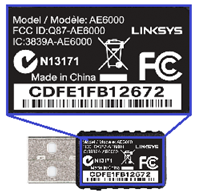Step 1:
Look at the back panel of the device.

Step 2:
You can find the model and serial number on the sticker at the back.
If there is no version number beside the model number, this means it is version 1.  In the example below, the model number is WVC54GC.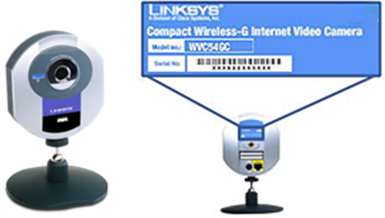Step 1:
Flip the device over.

Step 2:

You can find the model and serial number on the sticker underneath the device.  If there is no version number beside the model number, this means it is version 1.  In the example below, the model number of the device is BEFSR41 ver.4.1.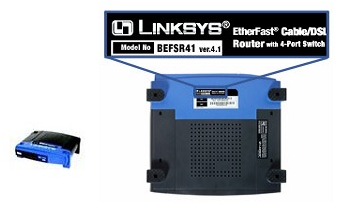Step 1:

Step 2:

You can find the model and serial number on a sticker on the device.  If there is no version number beside the model number, this means it is version 1.  In the example below, the model number is LNE100TX VERSION 4.1.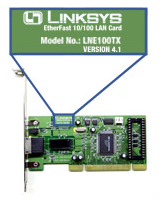Step 1:
Look at the front panel.

Step 2:

You can find the model number under the Linksys logo, on the upper-left side.  If there is no version number beside the model number, this means it is version 1.  In the example below, the model number of the device is SE3024.

NOTE:  The serial number is located at the back of your device.  For SE4008, you can find the model number underneath the device.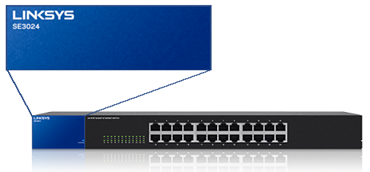SE Switches (Black)

Step 1:
Look at the top of the device.

Step 2:

You can find the model number below or next to the logo.  If there is no version number beside the model number, this means it is version 1.  In the example below, the model number of the device is SE1500.

NOTE:  The serial number is located underneath the device.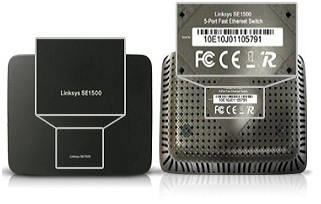Step 1:
Look at the front panel.

Step 2:

You can find the model number below the Linksys logo.  If there is no version number beside the model number, this means it is version 1.  In the example below, the model number of the device is WUMC710.

NOTE:  For older Linksys wireless bridges, you can find the model number and MAC address underneath the device.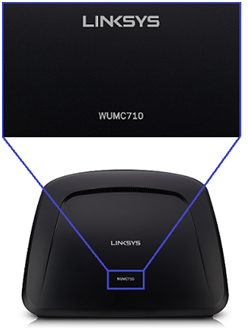Step 1:
Flip the device over.

Step 2:

You can find the model and serial number on the sticker underneath the device.  If there is no version number beside the model number, this means it is version 1.  In the example below, the model number is NMH410.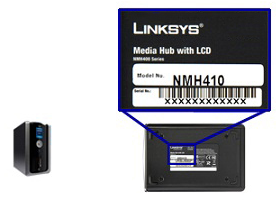Step 1:
Flip the device over.

Step 2:

You can find the model, MAC address, and serial number on the sticker underneath the device.  If there is no version number beside the model number, this means it is version 1.  In the example below, the model number of the device is DMC250.